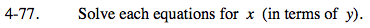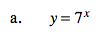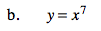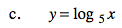### Home > PC > Chapter 4 > Lesson 4.2.1 > Problem4-77

4-77.
1. Solve each equations for x (in terms of y). Homework Help ✎

1. y = 7x

2. y = x7

3. y = log5 xTake the log of both sides.Take the 7th root of both sides.Rewrite the equation in exponential form.